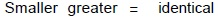# User Forum

Subject :IMO    Class : Class 5

Fill in the blanks and select the correct option.
(i) A fraction in which numerator is __P__ than the denominator is called a proper fraction.
(ii) All the factors of a number are __Q__ than or equal to the number.
(iii) Compare : 0.75 __R__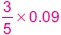(iv) The line along which a figure is folded such that two parts of the figure are __S__ is called the line of symmetry.

A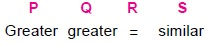B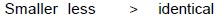C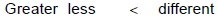D